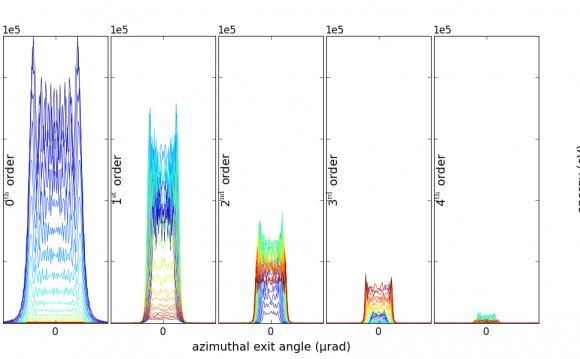# Diffraction grating derivation

February 11, 2022Diffraction is a process in which a collimated beam of radiation spreads out as it passes (1) though a narrow opening or (2) by a sharp barrier. An interesting distraction effect was observed in a laboratory setting by Thomas Young in 1801 when he performed the two-slit experiment (discussed below). Because diffraction depends on the wavelength of light, it can be used to separate polychromatic light (white light) into its constituent optical frequencies; in the case of white light, this would result in a rainbow of colors. The manipulation of diffraction forms the basis of selecting a narrow range of wavelengths of light in a monochromator.
In order to more easily understand how diffraction can be used to disperse wavelengths, picture the passage of radiation through a narrow barrier. Figure 1-7 illustrates such a barrier. As the slit width narrows waves of radiation spread out more strongly. The Heisenberg Uncertainty Principle indicates that as the uncertainty in the position of a photon decreases (in a direction transverse to its propagation direction), the uncertainty in its transverse momentum must increase; this uncertainty in transverse momentum is what causes the light to spread out. When the slit is sufficiently wide, the diffraction is small because there is still great uncertainty in position (Figure 1-7a). As the slit becomes more narrow (Figure 1-7b), the uncertainty in position becomes smaller which results in greater diffraction.

Figure 1-7. Variation in Slit Width Openings.

Diffraction is intimately related to the constructive and destructive interference of waves. Start by considering monochromatic electromagnetic radiation passing through two slits (Figure 1-8). The monochromatic light is passing through one narrow slit before being passed through two more slits. As radiation is passes through the second slits, diffraction occurs at both slits creating two beams that spread out and overlap. Constructive and deconstructive interference between these beams can be observed on a flat plate (a detector in this case). Regions on the detector in which the path length difference between these two waves is an integer multiple of the wavelengths constructively interfere and produce a large intensity. Positions on the detection plate that correspond to odd integer multiples of half a wavelength create deconstructive interference, with no resulting intensity. Overall the intensity distribution on the detector varies sinusoidally, with high intensity corresponding to constructive interference, and zero intensity corresponding to destructive interference. Each of these situations is illustrated in Figure 1-8. If the two slits are replaced by many narrow, equally spaced slits (i.e. a diffraction grating) the regions of constructive interference become very narrow. Instead of a sinusoidal variation in intensity, a series of narrow bright bands appears on the detector.

Figure 1-8. An Idealized Illustration of the Two-Slit Experiment Illustrating the Constructive and Deconstructive Nature of Electromagnetic Radiation. Distinct bands are show here for illustrative purposes only; the bands are actually broader areas of light (constructive interference) that gray to areas with no light (deconstructive interference). The blue lines above correspond to the alignment of maximum constructive interference.

The above discussion was for monochromatic radiation. If the concepts in Figure 1-8 are extended one step further and polychromatic light is used, the different colors of radiation are separated. All of the different wavelengths will constructively interfere in the center of the detector. However, since each color has a specific wavelength, the locations of the other interference maxima depend on the wavelength. As a result, two “rainbow” spectra of light will be centered around the top and bottom constructive interference lines shown in Figure 1-8.

While the diffractive properties of multiple transmissive slits could be utilized in analytical instruments, this would result in the creation of very narrow, but long instruments. As a result, mirrors that also create diffraction are utilized to make instruments more compact. They do this by bending light at a sharp angle, as observed below in the case of reflection grating monochromators. The principle is the same as a transmission grating; each of the sawtooth shaped steps in Figure 1-9 (referred to as a blazed surface) acts as a “slit”. Waves are diffracted off of each of these slits, and wavelength dependent constructive and destructive interference creates a rainbow spectrum in reflection. Monochromatic light is used for illustration purposes here to lessen the complexity of the figure. However the principles discussed below for a single wavelength apply to a mixture of wavelengths.

Figure 1-9 Diffraction Resulting from a Echellette (Conventional) Grating.

Typically radiant waves constructively interfere in two directions (+1 and -1) depending on the incident angle of incoming radiation and the grating spacing d. These two directions are analogous to the upper and lower constructive interference bands in Figure 1-8. There is one more complication that has been added to Figure 1-9, in Figure 1-8 incident radiation was perpendicular to the slits (parallel to the grating normal), whereas in Figure 1-9 incident radiation makes an angle i with respect to the grating normal (the dashed vertical line in Figure 1-9). Radiation will be diffracted to the left and to the right of the grating normal; these will be mathematically characterized below separately for two types of grating surfaces. The left-of-normal diffraction will be discussed first (the left-hand figure). The lower right-hand diagonal lines 1 and 2 are incoming monochromatic radiation and the upper right-hand diagonal lines 1’ and 2’ are the diffracted monochromatic radiation.

Source: people.whitman.edu